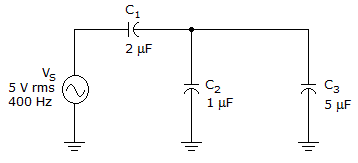# Electronics - Capacitors - Discussion

### Discussion :: Capacitors - Filling the Blanks (Q.No.14)

14.The reactance of C2 is _____ in the given circuit.

 [A]. 66[B]. 80[C]. 199[D]. 398Explanation:

No answer description available for this question.

 Jai said: (Jan 8, 2012) Xc=1/2*pi*f*c; xc=200ohm... How its 398 ohm?

 Jagat said: (Aug 20, 2012) = 1 / 2*3.14*400*1*10^-6.... (C2= 1 micro farad) = 1/0.0025136

 Ankita Upadhyay said: (Apr 26, 2013) Total c=(2*(5+1))/(2+5+1) = 1.5. R(total) = 1/(2*3.14*1.5*10^6) = 265.15. Now r1/(r2||r3) = (c2||c3)/c1. Therefore r1/(r2||r3) = 6/2 = 3. Therefore (r2||r3) = r/(r1+r2||r3) = 265.15/4 = 66.28. Now again r2/r3 = c3/c2. Thus r2 = (r2||r3)* (c3+c2)/c2 = 66.28 *6 /1 = 397.68 == 398.

 Kencha said: (Oct 11, 2016) Xc = 1/2 * pi * f * c. Xc = 1/ 2 * 3.14 * 400 * 1 * 10^-6, Xc = 10^6 / 2 * 3.14 * 400, Xc = 398.089, Xc = 398 ohm.